Updating search results...

# 73 Results

View
Selected filters:
• MCCRS.Math.Content.6.RP.A.1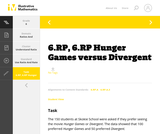Unrestricted Use
CC BY
Rating
0.0 stars

This is a task from the Illustrative Mathematics website that is one part of a complete illustration of the standard to which it is aligned. Each task has at least one solution and some commentary that addresses important aspects of the task and its potential use.

Subject:
Ratios and Proportions
Material Type:
Activity/Lab
Provider:
Illustrative Mathematics
Provider Set:
Illustrative Mathematics
Author:
Illustrative Mathematics
08/06/2015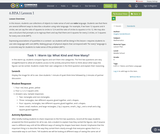Conditional Remix & Share Permitted
CC BY-NC
Rating
0.0 stars

In this lesson, students use collections of objects to make sense of and use ratio language. Students see that there are several different ways to describe a situation using ratio language. For example, if we have 12 squares and 4 circles, we can say the ratio of squares to circles is 12:4 and the ratio of circles to squares is 4 to 12. We may also see a structure that prompts us to regroup them and say that there are 6 squares for every 2 circles, or 3 squares for every one circle (MP7).Expressing associations of quantities in a context—as students will be doing in this lesson—requires students to use ratio language with care (MP6). Making groups of physical objects that correspond with “for every” language is a concrete way for students to make sense of the problem (MP1).

Subject:
Mathematics
Material Type:
Lesson Plan
Author:
Angela Vanderbloom
08/25/2018
Remix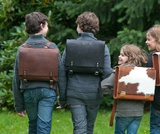Conditional Remix & Share Permitted
CC BY-NC
Rating
0.0 stars

Students work with a set of cards showing different ways of expressing ratios, including both part-part statements and part-whole statements. They group the cards that show the same ratio of boys to girls, but without the explicit use of the term equivalent.Key ConceptsRatios can be represented in a:b form, as fractions, as decimals, as factors, and in words; they can be expressed in part-part statements or in part-whole statements.Goals and Learning ObjectivesGroup cards showing ratios that are equivalent but expressed in different forms.

Subject:
Ratios and Proportions
Material Type:
Lesson Plan
Author:
Angela Vanderbloom
08/25/2018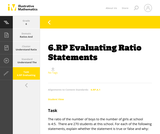Unrestricted Use
CC BY
Rating
0.0 stars

This is a task from the Illustrative Mathematics website that is one part of a complete illustration of the standard to which it is aligned. Each task has at least one solution and some commentary that addresses important aspects of the task and its potential use.

Subject:
Ratios and Proportions
Material Type:
Activity/Lab
Provider:
Illustrative Mathematics
Provider Set:
Illustrative Mathematics
Author:
Illustrative Mathematics
08/06/2015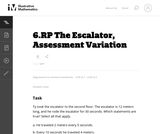Unrestricted Use
CC BY
Rating
0.0 stars

This is a task from the Illustrative Mathematics website that is one part of a complete illustration of the standard to which it is aligned. Each task has at least one solution and some commentary that addresses important asects of the task and its potential use. Here are the first few lines of the commentary for this task: Ty took the escalator to the second floor. The escalator is 12 meters long, and he rode the escalator for 30 seconds. Which statements are true? Select...

Subject:
Mathematics
Material Type:
Activity/Lab
Provider:
Illustrative Mathematics
Provider Set:
Illustrative Mathematics
Author:
Illustrative Mathematics
03/18/2013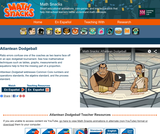Read the Fine Print
Some Rights Reserved
Rating
0.0 stars

Ratio errors confuse a dodgeball coach as two teams face off in an epic tournament. See how mathematical techniques such as tables, graphs, measurements and equations help to find the missing part of a proportion.

Subject:
Mathematics
Material Type:
Lecture
Provider:
Learning Games Lab
Author:
NMSU Learning Gams Lab
07/15/2015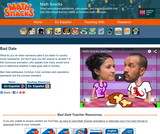Read the Fine Print
Some Rights Reserved
Rating
0.0 stars

True love has the right ratio. In this humorous animation, the number of words spoken by each partner predicts whether a date goes well or horribly. What do you do when someone asks if you listen to country music backwards, but won't let you get a word in edgewise?

Subject:
Mathematics
Material Type:
Lecture
Provider:
Learning Games Lab
Author:
NMSU Learning Games Lab
07/15/2015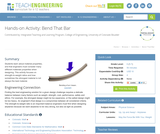Read the Fine Print
Educational Use
Rating
0.0 stars

Students learn about material properties, and that engineers must consider many different materials properties when designing. This activity focuses on strength-to-weight ratios and how sometimes the strongest material is not always the best material.

Subject:
Engineering
Physics
Material Type:
Activity/Lab
Provider:
TeachEngineering
Provider Set:
TeachEngineering
Author:
Alex Conner
Geoffrey Hill
Janet Yowell
Malinda Schaefer Zarske
Tom Rutkowski
10/14/2015Read the Fine Print
Educational Use
Rating
0.0 stars

Bianca visits a bike shop and learns how bicycle gears work in this Cyberchase video segment.

Subject:
Mathematics
Material Type:
Lecture
Provider:
PBS LearningMedia
Provider Set:
PBS Learning Media: Multimedia Resources for the Classroom and Professional Development
Author:
U.S. Department of Education
WNET
09/09/2008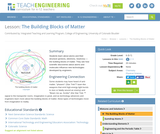Read the Fine Print
Educational Use
Rating
0.0 stars

Students learn about atoms and their structure (protons, electrons, neutrons) — the building blocks of matter. They see how scientific discoveries about atoms and molecules influence new technologies developed by engineers.

Subject:
Physical Science
Chemistry
Material Type:
Lesson
Provider:
TeachEngineering
Provider Set:
TeachEngineering
Author:
Brian Kay
Daria Kotys-Schwartz
Janet Yowell
Malinda Schaefer Zarske
02/17/2017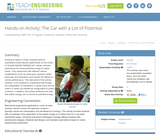Read the Fine Print
Educational Use
Rating
0.0 stars

Working in teams of three, students perform quantitative observational experiments on the motion of LEGO MINDSTORMS(TM) NXT robotic vehicles powered by the stored potential energy of rubber bands. They experiment with different vehicle modifications (such as wheel type, payload, rubber band type and lubrication) and monitor the effects on vehicle performance. The main point of the activity, however, is for students to understand that through the manipulation of mechanics, a rubber band can be used in a rather non-traditional configuration to power a vehicle. In addition, this activity reinforces the idea that elastic energy can be stored as potential energy.

Subject:
Engineering
Chemistry
Material Type:
Activity/Lab
Provider:
TeachEngineering
Provider Set:
TeachEngineering
Author:
Brian Kay
Daria Kotys-Schwartz
Janet Yowell
Malinda Schaefer Zarske
09/18/2014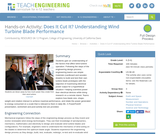Read the Fine Print
Educational Use
Rating
0.0 stars

Students gain an understanding of the factors that affect wind turbine operation. Following the steps of the engineering design process, engineering teams use simple materials (cardboard and wooden dowels) to build and test their own turbine blade prototypes with the objective of maximizing electrical power output for a hypothetical situation—helping scientists power their electrical devices while doing research on a remote island. Teams explore how blade size, shape, weight and rotation interact to achieve maximal performance, and relate the power generated to energy consumed on a scale that is relevant to them in daily life. A PowerPoint® presentation, worksheet and post-activity test are provided.

Subject:
Career and Technical Education
Mathematics
Measurement and Data
Numbers and Operations
Physical Science
Material Type:
Activity/Lab
Provider:
TeachEngineering
Author:
Alexander Kon
02/07/2017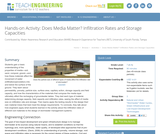Read the Fine Print
Educational Use
Rating
0.0 stars

Students gain a basic understanding of the properties of media soil, sand, compost, gravel and how these materials affect the movement of water (infiltration/percolation) into and below the surface of the ground. They learn about permeability, porosity, particle size, surface area, capillary action, storage capacity and field capacity, and how the characteristics of the materials that compose the media layer ultimately affect the recharging of groundwater tables. They test each type of material, determining storage capacity, field capacity and infiltration rates, seeing the effect of media size on infiltration rate and storage. Then teams apply the testing results to the design their own material mixes that best meet the design requirements. To conclude, they talk about how engineers apply what students learned in the activity about the infiltration rates of different soil materials to the design of stormwater management systems.

Subject:
Engineering
Hydrology
Material Type:
Activity/Lab
Provider:
TeachEngineering
Provider Set:
TeachEngineering
Author:
Brigith Soto
Jennifer Butler
Krysta Porteus
Maya Trotz
Ryan Locicero
William Zeman
09/18/2014Read the Fine Print
Educational Use
Rating
0.0 stars

Students are introduced to the concept of energy conversion, and how energy transfers from one form, place or object to another. They learn that energy transfers can take the form of force, electricity, light, heat and sound and are never without some energy "loss" during the process. Two real-world examples of engineered systems light bulbs and cars are examined in light of the law of conservation of energy to gain an understanding of their energy conversions and inefficiencies/losses. Students' eyes are opened to the examples of energy transfer going on around them every day. Includes two simple teacher demos using a tennis ball and ball bearings. A PowerPoint(TM) presentation and quizzes are provided.

Subject:
Engineering
Physics
Material Type:
Lesson Plan
Provider:
TeachEngineering
Provider Set:
TeachEngineering
Author:
Eric Anderson
Irene Zhao
Jeff Kessler
10/14/2015Read the Fine Print
Educational Use
Rating
0.0 stars

Students learn about kinetic and potential energy, including various types of potential energy: chemical, gravitational, elastic and thermal energy. They identify everyday examples of these energy types, as well as the mechanism of corresponding energy transfers. They learn that energy can be neither created nor destroyed and that relationships exist between a moving object's mass and velocity. Further, the concept that energy can be neither created nor destroyed is reinforced, as students see the pervasiveness of energy transfer among its many different forms. A PowerPoint(TM) presentation and post-quiz are provided.

Subject:
Engineering
Physics
Material Type:
Lesson Plan
Provider:
TeachEngineering
Provider Set:
TeachEngineering
Author:
Eric Anderson
Irene Zhao
Jeff Kessler
10/14/2015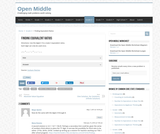Conditional Remix & Share Permitted
CC BY-NC-SA
Rating
0.0 stars

Open middle problems require a higher depth of knowledge than most problems that assess procedural and conceptual understanding. They support the Common Core State Standards and provide students with opportunities for discussing their thinking.

The Finding Equivalent Ratios problem asks students to use the digits 1-9 to create 3 equivalent ratios made up of single and double digit numbers.

Subject:
Mathematics
Material Type:
Activity/Lab
Provider:
Open Middle
08/21/2017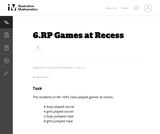Unrestricted Use
CC BY
Rating
0.0 stars

While students need to be able to write sentences describing ratio relationships, they also need to see and use the appropriate symbolic notation for ratios. If this is used as a teaching problem, the teacher could ask for the sentences as shown, and then segue into teaching the notation. It is a good idea to ask students to write it both ways (as shown in the solution) at some point as well.

Subject:
Mathematics
Material Type:
Activity/Lab
Provider:
Illustrative Mathematics
Provider Set:
Illustrative Mathematics
Author:
Illustrative Mathematics
05/01/2012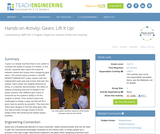Read the Fine Print
Educational Use
Rating
0.0 stars

A gear is a simple machine that is very useful to increase the speed or torque of a wheel. In this activity, students learn about the trade-off between speed and torque when designing gear ratios. The activity setup includes a LEGO(TM) MINDSTORMS(TM) NXT pulley system with two independent gear sets and motors that spin two pulleys. Each pulley has weights attached by string. In a teacher demonstration, the effect of adding increasing amounts of weight to the pulley systems with different gear ratios is observed as the system's ability to lift the weights is tested. Then student teams are challenged to design a gear set that will lift a given load as quickly as possible. They test and refine their designs to find the ideal gear ratio, one that provides enough torque to lift the weight while still achieving the fastest speed possible.

Subject:
Engineering
Material Type:
Activity/Lab
Provider:
TeachEngineering
Provider Set:
TeachEngineering
Author:
Jeffrey Laut
Paul Phamduy
10/14/2015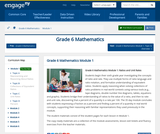Conditional Remix & Share Permitted
CC BY-NC-SA
Rating
0.0 stars

Students begin their sixth grade year investigating the concepts of ratio and rate. They use multiple forms of ratio language and ratio notation, and formalize understanding of equivalent ratios. Students apply reasoning when solving collections of ratio problems in real world contexts using various tools (e.g., tape diagrams, double number line diagrams, tables, equations and graphs). Students bridge their understanding of ratios to the value of a ratio, and then to rate and unit rate, discovering that a percent of a quantity is a rate per 100. The 35 day module concludes with students expressing a fraction as a percent and finding a percent of a quantity in real world concepts, supporting their reasoning with familiar representations they used previously in the module.

Subject:
Ratios and Proportions
Material Type:
Module
Provider:
New York State Education Department
Provider Set:
EngageNY
05/14/2013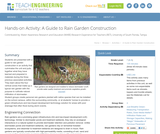Read the Fine Print
Educational Use
Rating
0.0 stars

Students are presented with a guide to rain garden construction in an activity that culminates the unit and pulls together what they have learned and prepared in materials during the three previous associated activities. They learn about the four vertical zones that make up a typical rain garden with the purpose to cultivate natural infiltration of stormwater. Student groups create personal rain gardens planted with native species that can be installed on the school campus, within the surrounding community, or at students' homes to provide a green infrastructure and low-impact development technology solution for areas with poor drainage that often flood during storm events.

Subject:
Engineering
Hydrology
Material Type:
Activity/Lab
Provider:
TeachEngineering
Provider Set:
TeachEngineering
Author:
Brigith Soto
Jennifer Butler
Krysta Porteus
Maya Trotz
Ryan Locicero
William Zeman
09/18/2014Conditional Remix & Share Permitted
CC BY-NC-SA
Rating
0.0 stars

In this activity, learners use their hands as tools for indirect measurement. Learners explore how to use ratios to calculate the approximate height of something that can't be measured directly by first measuring something that can be directly measured. This activity can also be used to explain how scientists use indirect measurement to determine distances between things in the universe that are too far away, too large or too small to measure directly (i.e. diameter of the moon or number of bacteria in a volume of liquid).

Subject:
Mathematics
Material Type:
Activity/Lab
Provider:
Exploratorium
Author:
Exploratorium
Gordon and Betty Moore Foundation
National Science Foundation
The Exploratorium
12/07/2010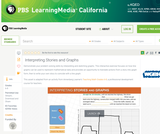Read the Fine Print
Educational Use
Rating
0.0 stars

In this interactive activity adapted from Annenberg Learner's Teaching Math Grades 6&ndash;8, explore some of the ways graphs can represent mathematical data contained in a story.

Subject:
Mathematics
Material Type:
Interactive
Provider:
PBS LearningMedia
Provider Set:
PBS Learning Media Common Core Collection
Author:
U.S. Department of Education
WGBH Educational Foundation
06/20/2012Unrestricted Use
CC BY
Rating
0.0 stars

This lesson is regarding the introduction to ratios.  Students will learn what a ratio is, practice finding ratios, and work on making their own ratios. There are YouTube videos, worksheets, and funny images to help students understand ratios.

Subject:
Mathematics
Ratios and Proportions
Material Type:
Assessment
Homework/Assignment
Interactive
Author:
Maggie Davenport
07/07/2021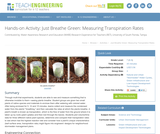Read the Fine Print
Educational Use
Rating
0.0 stars

Through multi-trial experiments, students are able to see and measure something that is otherwise invisible to them seeing plants breathe. Student groups are given two small plants of native species and materials to enclose them after watering with colored water. After being enclosed for 5, 10 and 15 minutes, teams collect and measure the condensed water from the plants' "breathing," and then calculate the rates at which the plants breathe. A plant's breath is known as transpiration, which is the flow of water from the ground where it is taken up by roots (plant uptake) and then lost through the leaves. Students plot volume/time data for three different native plant species, determine and compare their transpiration rates to see which had the highest reaction rate and consider how a plant's unique characteristics (leaf surface area, transpiration rate) might figure into engineers' designs for neighborhood stormwater management plans.

Subject:
Engineering
Hydrology
Material Type:
Activity/Lab
Provider:
TeachEngineering
Provider Set:
TeachEngineering
Author:
Brigith Soto
Jennifer Butler
Krysta Porteus
Maya Trotz
Ryan Locicero
William Zeman
09/18/2014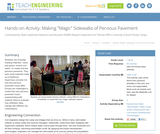Read the Fine Print
Educational Use
Rating
0.0 stars

Students use everyday building materials sand, pea gravel, cement and water to create and test pervious pavement. They learn what materials make up a traditional, impervious concrete mix and how pervious pavement mixes differ. Groups are challenged to create their own pervious pavement mixes, experimenting with material ratios to evaluate how infiltration rates change with different mix combinations.

Subject:
Engineering
Material Type:
Activity/Lab
Provider:
TeachEngineering
Provider Set:
TeachEngineering
Author:
Brigith Soto
Jennifer Butler
Krysta Porteus
Maya Trotz
Ryan Locicero
William Zeman
09/18/2014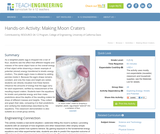Read the Fine Print
Educational Use
Rating
0.0 stars

As a weighted plastic egg is dropped into a tub of flour, students see the effect that different heights and masses of the same object have on the overall energy of that object while observing a classic example of potential (stored) energy transferred to kinetic energy (motion). The plastic egg's mass is altered by adding pennies inside it. Because the egg's shape remains constant, and only the mass and height are varied, students can directly visualize how these factors influence the amounts of energy that the eggs carry for each experiment, verified by measurement of the resulting impact craters. Students learn the equations for kinetic and potential energy and then make predictions about the depths of the resulting craters for drops of different masses and heights. They collect and graph their data, comparing it to their predictions, and verifying the relationships described by the equations. This classroom demonstration is also suitable as a small group activity.

Subject:
Engineering
Physics
Material Type:
Activity/Lab
Provider:
TeachEngineering
Provider Set:
TeachEngineering
Author:
Eric Anderson
Irene Zhao
Jeff Kessler
10/14/2015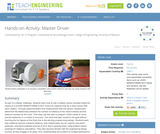Read the Fine Print
Educational Use
Rating
0.0 stars

As part of a design challenge, students learn how to use a rotation sensor (located inside the casing of a LEGO® MINDSTORMS ® NXT motor) to measure how far a robot moves with each rotation. Through experimentation and measurement with the sensor, student pairs determine the relationship between the number of rotations of the robot's wheels and the distance traveled by the robot. Then they use this ratio to program LEGO robots to move precise distances in a contest of accuracy. The robot that gets closest to the goal without touching the toy figures at the finish line is the winning programming design. Students learn how rotational sensors measure distance, how mathematics can be used for real-world purposes, and about potential sources of error due to gearing when using rotation sensor readings for distance calculations. They also become familiar with the engineering design process as they engage in its steps, from understanding the problem to multiple test/improve iterations to successful design.

Subject:
Engineering
Electronic Technology
Material Type:
Activity/Lab
Provider:
TeachEngineering
Provider Set:
TeachEngineering
Author:
Nishant Sinha
Pranit Samarth
Satish S. Nair
09/18/2014Conditional Remix & Share Permitted
CC BY-NC
Rating
0.0 stars

Subject:
Mathematics
Material Type:
Full Course
Provider:
Pearson
10/06/2016Conditional Remix & Share Permitted
CC BY-NC
Rating
0.0 stars

Students work in a whole-class setting, independently, and with partners to design and implement a problem-solving plan based on the mathematical concepts of rates and multiple representations (e.g., tables, equations, and graphs). They analyze a rule of thumb and use this relationship to calculate the distance in miles from a viewer's vantage point to lightning.Key ConceptsThroughout this unit, students are encouraged to apply the mathematical concepts they have learned over the course of this year to new settings. Help students develop and refine these problem-solving skills:Creating a problem-solving plan and implementing the plan systematicallyPersevering through challenging problems to find solutionsRecalling prior knowledge and applying that knowledge to new situationsMaking connections between previous learning and real-world problemsCommunicating their approaches with precision and articulating why their strategies and solutions are reasonableCreating efficacy and confidence in solving challenging problems in the real worldGoals and Learning ObjectivesCreate and implement a problem-solving plan.Organize and interpret data presented in a problem situation.Analyze the relationship between two variables.Create a rate table to organize data and make predictions.Apply the relationship between the variables to write a mathematical formula and use the formula to solve problems.Create a graph to display proportional relationships, and use this graph to make predictions.Articulate strategies, thought processes, and approaches to solving a problem, and defend why the solution is reasonable.

Subject:
Algebra
Material Type:
Lesson Plan
Provider:
Pearson
09/21/2015Rating
0.0 stars

Ratios

Type of Unit: Concept

Prior Knowledge

Students should be able to:

Calculate with whole numbers up to 100 using all four operations.
Understand fraction notation and percents and translate among fractions, decimal numbers, and percents.
Interpret and use a number line.
Use tables to solve problems.
Use tape diagrams to solve problems.
Sketch and interpret graphs.
Write and interpret equations.

Lesson Flow

The first part of the unit begins with an exploration activity that focuses on a ratio as a way to compare the amount of egg and the amount of flour in a mixture. The context motivates a specific understanding of the use of, and need for, ratios as a way of making comparisons between quantities. Following this lesson, the usefulness of ratios in comparing quantities is developed in more detail, including a contrast to using subtraction to find differences. Students learn to interpret and express ratios as fractions, as decimal numbers, in a:b form, in words, and as data; they also learn to identify equivalent ratios.

The focus of the middle part of the unit is on the tools used to represent ratio relationships and on simplifying and comparing ratios. Students learn to use tape diagrams first, then double number lines, and finally ratio tables and graphs. As these tools are introduced, students use them in problem-solving contexts to solve ratio problems, including an investigation of glide ratios. Students are asked to make connections and distinctions among these forms of representation throughout these lessons. Students also choose a ratio project in this part of the unit (Lesson 8).

The third and last part of the unit covers understanding percents, including those greater than 100%.

Students have ample opportunities to check, deepen, and apply their understanding of ratios, including percents, with the selection of problems in the Gallery.

Subject:
Mathematics
Statistics and Probability
Material Type:
Unit of Study
Provider:
Pearson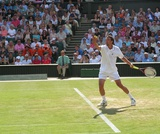Conditional Remix & Share Permitted
CC BY-NC
Rating
0.0 stars

This lesson formally introduces and defines a ratio as a way of comparing numbers to one another.Key ConceptsA ratio is defined by the following characteristics:A ratio is a pair of numbers (a:b).Ratios are used to compare two numbers.The value of a ratio a:b is the quotient a ÷ b, or the result of dividing a by b.Other important features of ratios include the following:A ratio does not always tell you the values of quantities being compared.The order of values in a ratio matters.Goals and Learning ObjectivesIntroduce a formal definition of ratio.Use the definition of ratio to solve problems related to comparing quantities.Understand that ratios do not always tell you the values of the quantities being compared.Understand that the order of values in a ratio matters.

Subject:
Ratios and Proportions
Material Type:
Lesson Plan
Provider:
Pearson
09/21/2015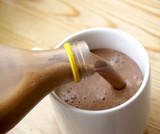Conditional Remix & Share Permitted
CC BY-NC
Rating
0.0 stars

Students watch a video in which a double number line is used to solve a problem about getting the right amount of protein mix. Using the double number line is an example of modeling with mathematics, which is Mathematical Practice 4.Key ConceptsA double number line shows corresponding values for two variable quantities with a constant ratio between them. Each pair of tick marks that go together shows a ratio equivalent to all of the other ratios between corresponding tick marks.Goals and Learning ObjectivesWatch an example of students using mathematics to model a relationship between quantities (MP4).Use a double number line to solve a problem.Use a double number line to deepen understanding of equivalence in the context of a relationship between quantities with a constant ratio.SWD: Some students with disabilities will benefit from a preview of the goals in each lesson. Students can highlight the critical features and/or concepts and will help them to pay close attention to salient information.

Subject:
Ratios and Proportions
Material Type:
Lesson Plan
Provider:
Pearson
09/21/2015Conditional Remix & Share Permitted
CC BY-NC
Rating
0.0 stars

Students use double number lines to model relationships and to solve ratio problems.Key ConceptsDouble number line diagrams are useful for visualizing ratio relationships between two quantities. They are best used when the quantities have different units. (The unit rate appears paired with 1.) Double number line diagrams help students more easily “see” that there are many equivalent forms of the same ratio.Goals and Learning ObjectivesUnderstand double number line diagrams as a way to visually compare two quantities.Use double number line diagrams to solve ratio problems.

Subject:
Ratios and Proportions
Material Type:
Lesson Plan
Provider:
Pearson
09/21/2015Conditional Remix & Share Permitted
CC BY-NC
Rating
0.0 stars

Students are asked to fix a botched mixture that does not follow a given recipe. To fix the mixture, students must find a ratio of eggs to flour that is equivalent to 2:3, but without explicit instruction on the concept of equivalent ratios.Key ConceptsStudents are invited to investigate the underlying idea of equivalent ratios by “correcting” the ratio between two ingredients in a botched mixture that does not follow a given recipe.Goals and Learning ObjectivesExplore a problem based on a recipe with two ingredients.Share approaches, clarify reasoning, and develop clear explanations of how to know a mixture has the right balance of ingredients.

Subject:
Ratios and Proportions
Material Type:
Lesson Plan
Provider:
Pearson
09/21/2015Conditional Remix & Share Permitted
CC BY-NC
Rating
0.0 stars

Students work with a set of cards showing different ways of expressing ratios, including both part-part statements and part-whole statements. They group the cards that show the same ratio of boys to girls, but without the explicit use of the term equivalent.Key ConceptsRatios can be represented in a:b form, as fractions, as decimals, as factors, and in words; they can be expressed in part-part statements or in part-whole statements.Goals and Learning ObjectivesGroup cards showing ratios that are equivalent but expressed in different forms.

Subject:
Ratios and Proportions
Material Type:
Lesson Plan
Provider:
Pearson
09/21/2015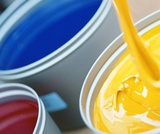Conditional Remix & Share Permitted
CC BY-NC
Rating
0.0 stars

Students work with a set of cards showing different ways of expressing ratios numerically. They group the cards showing equivalent ratios and then order the groups from least to greatest value.Key ConceptsIt can be hard to compare the values of ratios represented in different forms (e.g., a:b, decimal, fraction, a to b). Simplifying ratios makes it easier to compare and order their values.Goals and Learning ObjectivesIdentify ratios that are equivalent but expressed differently.Simplify ratios in order to group and order cards efficiently and successfully.

Subject:
Ratios and Proportions
Material Type:
Lesson Plan
Provider:
Pearson
09/21/2015Conditional Remix & Share Permitted
CC BY-NC
Rating
0.0 stars

Students use informal methods of their own choosing to find percents of randomly generated monetary values.Key ConceptsMany approaches work for solving percent problems. This lesson focuses on experimenting with a range of approaches and understanding why and how multiple approaches yield correct results.Goals and Learning ObjectivesFind a percent of a given quantity.Find a quantity given a part and the percent that part is of the whole.Use percents in money calculations.

Subject:
Ratios and Proportions
Material Type:
Lesson Plan
Provider:
Pearson
09/21/2015Conditional Remix & Share Permitted
CC BY-NC
Rating
0.0 stars

This lesson introduces the concept of a glide ratio and encourages students to use appropriate tools strategically (Mathematical Practice 5). Students use tape diagrams, double number lines, ratio tables, graphs, and equations to represent glide ratios.Key ConceptsA glide ratio for an object or an organism in flight is the ratio of forward distance to vertical distance (in the absence of power and wind). For a given object or organism that glides, this ratio has a constant value and is treated as a feature of the object or organism.Goals and Learning ObjectivesUnderstand the concept of a glide ratio.Make connections within and between different ways of representing ratios.

Subject:
Ratios and Proportions
Material Type:
Lesson Plan
Provider:
Pearson
09/21/2015Conditional Remix & Share Permitted
CC BY-NC
Rating
0.0 stars

Students interpret multiple categories of data about a hypothetical village population that represents the global population. They determine whether percent statements about the data are true or false.Key ConceptsData presented in multiple formats can be investigated using percent statements that facilitate comparisons between different parts of a whole. In using percents to interpret data, it is essential to be clear about what the part is and what the whole is. The whole in this lesson is a representative sample of the global population, which is used as a model for investigating variation across the population.Goals and Learning ObjectivesInterpret data presented in different formats in terms of percents.Identify percent statements as true or false, if possible, and explain the decision.Modify false percents statements to make them true.

Subject:
Ratios and Proportions
Material Type:
Lesson Plan
Provider:
Pearson
09/21/2015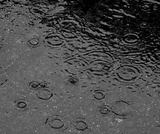Conditional Remix & Share Permitted
CC BY-NC
Rating
0.0 stars

Students use percents greater than 100% to solve problems about rainfall, revenue, snowfall, and school attendance.Key ConceptsPercents greater than 100% are useful in making comparisons between the values of a single quantity at two points in time. When a later value is more than 100% of an earlier value, it means the quantity has increased over time. This percent comparison can be used to find unknown values, whether the earlier or later value is unknown.Goals and Learning ObjectivesUnderstand the meaning of a percent greater than 100% in real-world situations.Use percents greater than 100% to interpret situations and solve problems.

Subject:
Ratios and Proportions
Material Type:
Lesson Plan
Provider:
Pearson